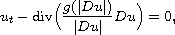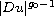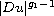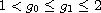Electron. J. Diff. Equ., Vol. 2015 (2015), No. 288, pp. 1-24.

### Holder continuity of bounded weak solutions to generalized parabolic p-Laplacian equations II: singular case Sukjung Hwang, Gary M. Lieberman

Abstract:
Here we generalize quasilinear parabolic p-Laplacian type equations to obtain the prototype equationwhere g is a nonnegative, increasing, and continuous function trapped in between two power functionsandwith. Through this generalization in the setting from Orlicz spaces, we provide a uniform proof with a single geometric setting that a bounded weak solution is locally Holder continuous with some degree of commonality between degenerate and singular types. By using geometric characters, our proof does not rely on any of alternatives which is based on the size of solutions.

Submitted July 17, 2015. Published November 19, 2015.
Math Subject Classifications: 35B45, 35K67.
Key Words: Quasilinear parabolic equation; singular equation; generalized structure; a priori estimate; Holder continuity.

Show me the PDF file (343 KB), TEX file, and other files for this article.Sukjung Hwang Center for Mathematical Analysis and Computation Yonsei University, Seoul 03722, Korea email: sukjung_hwang@yonsei.ac.kr Gary M. Lieberman Department of Mathematics Iowa State University Ames, IA 50011, USA email: lieb@iastate.edu